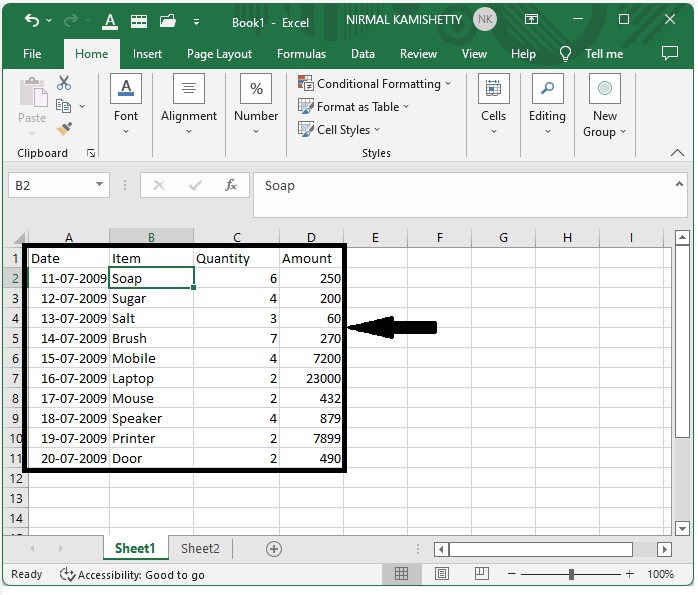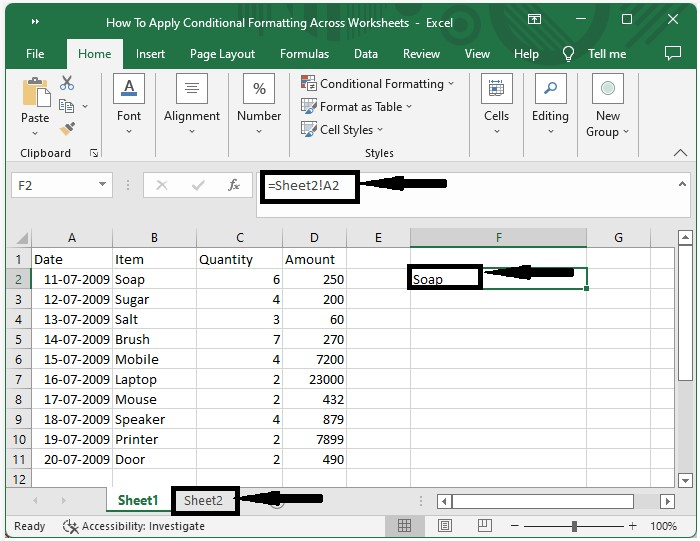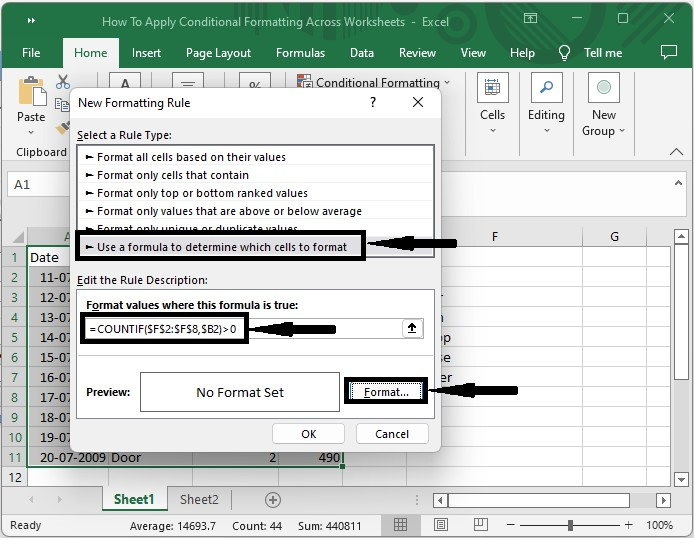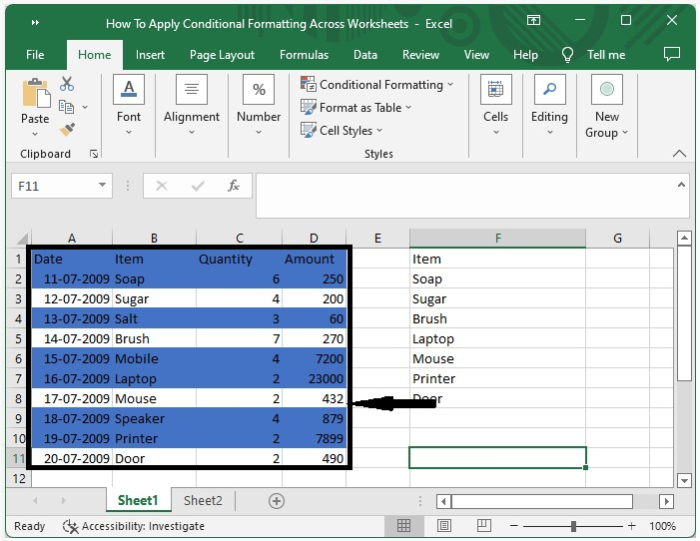# How to Apply Conditional Formatting across Worksheets /Workbooks in Excel?

You could have used conditional formatting in Excel very frequently, but have you ever tried applying conditional formatting across different work sheets in Excel? Yes, it is possible to apply conditional formatting across worksheets or workbooks in Excel; it is a very simple process. This tutorial will help you understand how we can apply conditional formatting across worksheets in Excel.

## Applying Conditional Formatting across Worksheets/Workbooks

Here, we will first get the data into one sheet and then apply the conditional formatting to the acquired data. Let's look at a quick way to apply conditional formatting to multiple worksheets in Excel.

Step 1

Let us consider that we have an Excel workbook whose data is similar to the data shown in the below figure.Now we need to format the data in img1 using the data present in img2.

To access data from multiple workbooks at the same time, ensure that the excel file is saved on the computer.

Now click on an empty cell and enter the formula =Sheet2!A2 if the data is present in the same workbook, and click on OK to copy the data into one sheet.Then drag down until all the results are copied into one workbook.

Step 2

Select the data to be formatted and then select a new rule under conditional formatting in the Excel ribbon; a new pop-up window will appear, as shown in the image below.Now in the new pop-up window, click on "Use a Formula" and enter the formula as

=COUNTIF($F$2:$F$8,\$B2)>0 in the formula box, then click on Format and select the colour from the new popup, then click OK to close the popup, and our final output will look like the below image.## Conclusion

In this tutorial, we used a simple example to demonstrate how you can apply conditional formatting across worksheets and workbooks in Excel to highlight a particular set of data.

Updated on: 03-Jan-2023

842 Views